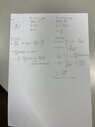# Speed of Current in a coaxial cable

Kyuubi
Homework Statement:
Calculate the inductance and capacitance per unit length of a long coaxial cable, and show that the current, or equivalently charge, wave has speed v =1/√(µ0ϵ0) = c which is the speed of light.
Relevant Equations:
Maxwell's equation in a vacuum.
Excuse me for not writing them, I'm not familiar with latex and I think I'll only need a conceptual answer. They should be in the photo though.
I've found the inductance and capacitance per unit length in a long coaxial cable. I even clearly see that if I multiply the two, I can get the speed of light. How do I begin to find the current wave and its speed though?

#### Attachments

•IMG_8707.jpg
22.8 KB · Views: 15

•